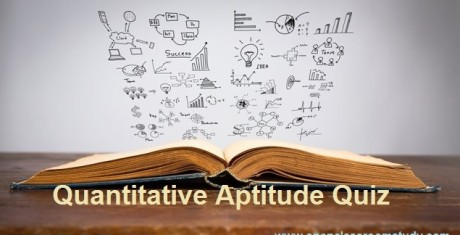# Mixed Aptitude Quiz - 7 : For IBPS PO Pre Exam 2016#### Ques 1.

0.875   2.75   7.5   18   40   85   176    ?

Solution :
0.875*2+1 = 2.75
2.75*2+2 = 7.5
7.5*2+3 = 18
18*2+4 = 40
40*2 + 5 = 85
85*2+6 = 176
176*2+7 = 359

#### Ques 2.

5 men or 10 women can do a piece of work in 25 days.How long will 10 men and 30 women take to finish the work?

Solution :

Time take to finish work = 25(10/5 + 30/10) = 5 days

#### Ques 3.

I. 72×^2 + x – 1 = 0         II. 63y^2 – 25y + 2 = 0

Solution :
==> 72x^2+9x-8x-1=0
==> 9x(8x+1)-1(8x+1)=0
==>(9x-1)(8x+1) = 0
==> X = 1/9 and -1/8

==>63y^2-14y-9y+2=0
==>7y(9y-2)-1(9y-2)=0
==> (7y-1)(9y-2)=0
==> Y = 1/7 and 2/9
x ≤ y

#### Ques 4.

(4/7)×2126÷11.4+26% of 520

Solution :
==> 0.57×186.5+135.2
==>106.3+135.2 = 241.5

#### Ques 5.

At what interest per annum will a sum of money double in 14 yrs ?

Solution :
SI = P and T = 14
SI = P*R*T/100
==>R = (100*P)/(P*14) = 100/14 = 7.14%

#### Ques 6.

The age of A is 54yrs, If B’s age is 36% more than that of A, What % is A’s age less than B?

Solution :

B age  = 136*54/100 = 73
A's age less than B = 73-54/73 *100= 26%

#### Ques 7.

The average age of  40 students in a class is 20 years.The average age of 30 students is 17.What is the average age of remaining students ?

Solution :
Total ages of 40 students = 40*20 =800
Total ages of 30 students = 30*17 = 510
Average of remaining = (800-510)/10 = 29years

#### Ques 8.

The area of a square field is 4050 mthe length of its diagonal is

Solution :
1/2 d2 = 4050
==>d2 = 8100
==. d = 90 m

#### Ques 9.

The milk and water in two vessels P and Q are in the ratio 4:3 and 2:3 respectively. In what ratio the liquids in both the vessels be mixed to obtain a new mixture in vessel R consisting half milk and half water?

Solution :

4/7                   2/5

1/2

1/10                 1/14

In R milk and water ratio = 1/10 : 1/14 = 7:5

#### Ques 10.

A bag contains 4 White shirts , 3 Red shirts and 5 Green shirts, if 2 shirts are drawn randomly, What is the probability both of them are White ?

Solution:
2 shirts drawn from 12 shirts 12C2 = 12*11/2 = 66
2 shirts drawn from 4 White shirts = 4C2 = 4*3/2 = 12/2 = 6
Probability of 2 shirts are white from 12 shirts  = 6/66 = 1/11